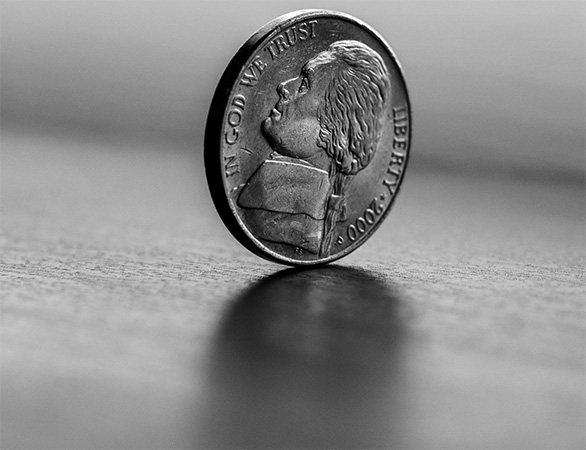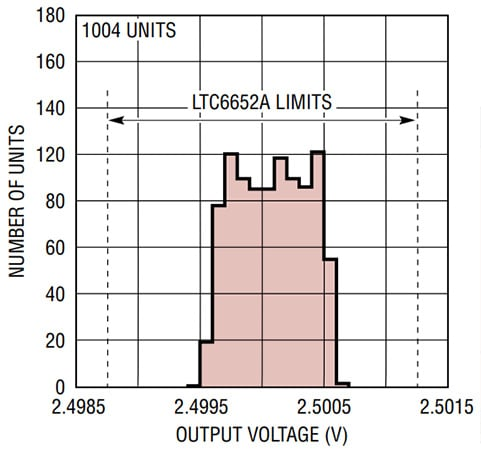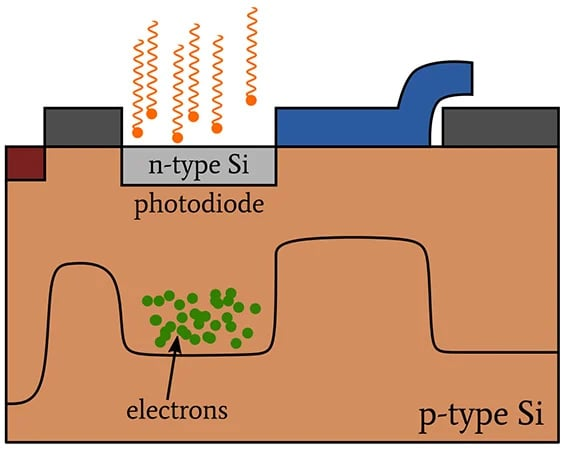Technical Article

# Introduction to Statistical Analysis in Electrical Engineering

June 09, 2020 by Robert Keim

## This article explores statistics and probability as tools that help us to understand circuit behavior and characterize engineered systems.

### Why We Use Statistics

Statistical techniques often don’t play a prominent role in circuit design, and fundamental circuit analysis is not statistical in nature; this makes sense because we take a deterministic approach when drawing schematics and calculating voltages or currents: we apply laws, theorems, equations, best practices, etc., and assume that these will result in accurate values and functional circuits.

The use of statistics and probability, on the other hand, is closely related to phenomena that humans cannot predict or control. We often express this idea using the word “random,” but as my university stats professor memorably pointed out, whether anything can be truly random is a philosophical question. When we’re working in the mathematical realm, the essential concept is that certain events and characteristics appear random or are effectively random.

Consider flipping a coin.##### Is it possible to determine—using physics—the side on which a flipped coin will land?

We discuss coin-toss results in terms of probability, but surely there is nothing random about this procedure. All we need is a computer program that considers the initial inclination of the coin, the contact point of the thumbnail, the amount of force applied while flipping, the density and composition of the air, the wind speed, convection currents, the elasticity of the surface on which the coin falls, and maybe a few dozen other variables, and we’ll be able to calculate whether the result will be heads or tails.

However, creating and fine-tuning this program would be approximately ten million times more difficult than adopting a statistical approach to coin tosses. This reminds us that sometimes it makes a lot of sense to assume that a phenomenon is random and then apply statistics.

### Statistics in Electrical Engineering

Even if we don’t need statistics when we’re designing a circuit or laying out a PCB, statistical methods are valuable when we need to characterize a circuit or system. Engineering organizations often cannot create a device, confirm that it “works,” and move on to the next project.

Rather, various questions may need to be investigated. We can turn to statistical analysis when these involve complex interactions or seemingly random phenomena; for example, what is the average signal-to-noise ratio across a sample of boards, and how much does SNR vary from one board to the next? Is there a correlation between ambient temperature and failure rate? What percentage of products will fall outside the acceptable range for battery life? Which operating conditions exhibit a statistically significant relationship with the bit error rate produced by an RF data link?##### Datasheets sometimes use statistics to convey information about performance parameters. Plot courtesy of Analog Devices.

Statistical analysis is particularly important in the field of IC research and development. Stochastic processes—i.e., procedures that require a statistical approach because they cannot be precisely predicted—become more prominent as feature sizes diminish. A research paper published in 2014 even introduced the concept of “stochastic electronics” as a new paradigm for the design of integrated circuits.

Signal processing also makes extensive use of statistics and probability. This is an expansive topic that far exceeds the scope of this article; for those interested, you can begin with a freely available, 475-page PDF textbook written by two professors: An Introduction to Statistical Signal Processing.

#### Noise

The fundamental physical processes that produce electrical noise operate in a way that is (as far as we can tell) random, and the resulting variations in voltage and current also appear to be random. Thus, it’s not surprising that we use statistical methods to measure and classify noise.

My colleague Stephen Colley has already written an excellent article on the statistical nature of noise, so I won’t dwell on the material that he has already covered. His article discusses the distribution of noise values and clearly explains the root-sum-square method for combining the amplitudes of uncorrelated noise sources.

An important aspect of noise analysis is rooted in the discrete nature of electric charge. Independent discrete events that occur in unpredictable ways are analyzed with the help of the Poisson distribution. For electrical engineers, probably the most critical mathematical relationship in Poisson statistics is the following: the noise level associated with a signal level is equal to the square root of the signal level. You can read more about this topic in my articles on CCD dark noise and CCD photon noise.The particle nature of light and of electric current both lead to shot noise, also called Poisson noise.

### Recap

Statistics and probability are closely related mathematical disciplines that help us to analyze, predict, and respond to phenomena that occur in a random or extremely complex fashion. Statistical techniques help us to characterize circuits and systems, perform digital signal processing, and deal with the omnipresent and perpetually troublesome electrical signals that we call noise.

In a future article, we’ll discuss specific statistical concepts that are relevant to the work of electrical engineers.•amiyasar January 16, 2021
•RK37 February 27, 2021